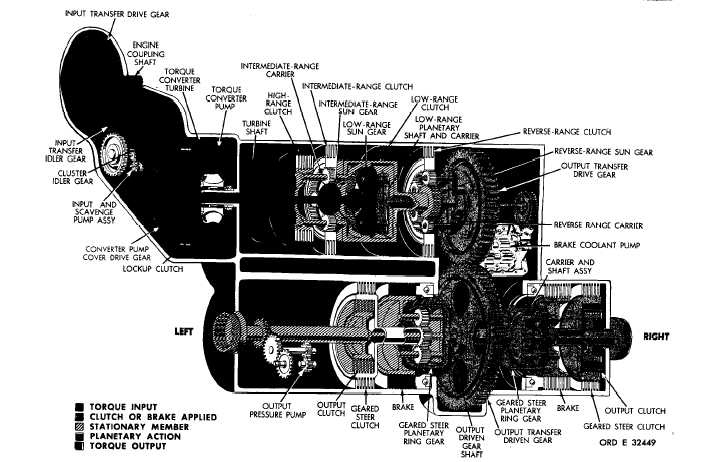Custom SearchP A R   5   4 T O R Q U E     P A T HS C H A P    2,   SEC  V Figure   30.   Second-gear,   full-left-steer   torque   path,   lockup   operation 54.   TORQUE   PATH - SECOND   GEAR, FULL - LEFT    STEER,    LOCKUP OPERATION   (fig.   30) a  .   T o r q u e    i s    t r a n s m i t t e d    t o    t h e    s t e er planetary   ring   gears   in   the   same   manner   as t h a t    f o r    f i r s t    g e a r ,    a s    d e s c r i b e d    i n    p a r .    4 7a a n d   ,   above.   From   this   point,   to   the   power train   outputs,   the   torque   paths   for   the   right and   left   sides   are   different. .   T h e    c l u t c h - b r a k e    m e t h o d    i s    u s e d    f or s e c o n d - g e a r    s t e e r .    I n    t h i s    m e t h o d ,    s t e e r i n g results   when   the   brake   is   applied   at   one   side while   the   drive   clutch   is   engaged   at   the   opposite side.   In   second   gear,   the   drive   clutch   is   the output   clutch. The   vehicle   steers   toward   the side   on   which   the   brake   is   applied. .   In   left   steer,   in   second   gear,   the   left brake   is   applied   while   the   left-output   clutch i s     r e l e a s e d. The   drive   clutch   (output   clutch) on   the   right   side   is   still   engaged   and   continues to   drive. Hydraulic   action,   controlled   by   the steer   control   valve,   releases   the   drive   clutch and   applies   the   brake.   An   opposite   movement o f    t h e    s t e e r    c o n t r o l    v a l v e    w i l l    r e l e a s e    t he right-output   clutch,   apply   the   right   brake,   and result   in   right   steer. .   Full   and   partial   steer   in   second   gear is   controlled   as   explained   in   par.   53d,   above. R e f e r    t o  T A B U L A T E D     D A T A ,    p a r .    7 ,    f or g e a r    r a t i o s    a p p l i c a b l e    t o    s e c o n d    g e a r ,    l e ft s t e e r. 5 7